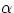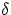Next: 5.4.4 Other Corrections Up: 5.4 Corrections for IUESIPS Previous: 5.4.2 Sensitivity Degradation Corrections

## 5.4.3 Heliocentric Velocity Correction

On 10 November 1981, IUESIPS (at Goddard) began to apply a correction for spacecraft motion to high dispersion spectra. This correction uses a set of orbital elements from 1979 to calculate IUE's contribution to the velocity vector. A correction using contemporaneous orbital elements may be calculated using the routine IUEVEL. Type:

IUEVEL,IMAGET,v,vnet,vdc

V is the vector velocity in right-handed rectangular equatorial coordinates, and therefore has three elements (V=[Vx,Vy,Vz]). Vnet is equal to the following:

VNET = Vxcoscos+ Vysincos+ Vzsinwhereandare the right ascension and declination of the object, respectively. VDC is equal to VNET divided by the speed of light. The wavelength correction can be done as follows:

wavecor = (1 + VDC) × wave

Do not forget to un-correct for the IUESIPS correction, if it was already applied. The net velocity corrected for will be shown at the bottom of the label, or in element 54 of your 'H' vector (divide H(54) by 10). Its units are kilometers per second, so divide it by the speed of light before proceeding.

Note that this procedure is not currently compatible with NEWSIPS data, which uses the most recent previous set of orbital elements. It is possible that users may wish to use the following set of elements instead (e.g., if there was a delta-V maneuver between the epoch of the previous set and the date of observation. Until IUEVEL is changed, you can work around this restriction by invoking it with a null string in place of the file name. You'll be prompted for the observation date (in Julian days!) and the target coordinates (in decimal degrees).Next: 5.4.4 Other Corrections Up: 5.4 Corrections for IUESIPS Previous: 5.4.2 Sensitivity Degradation Corrections

2/9/1998